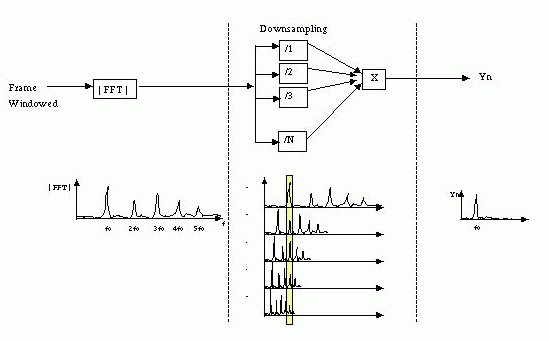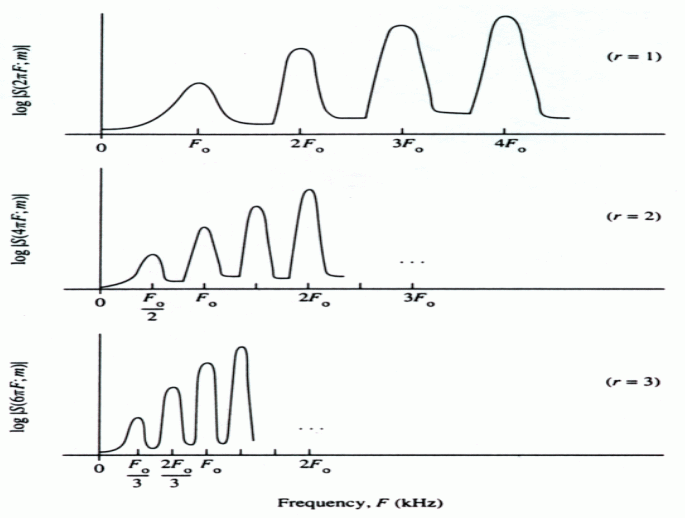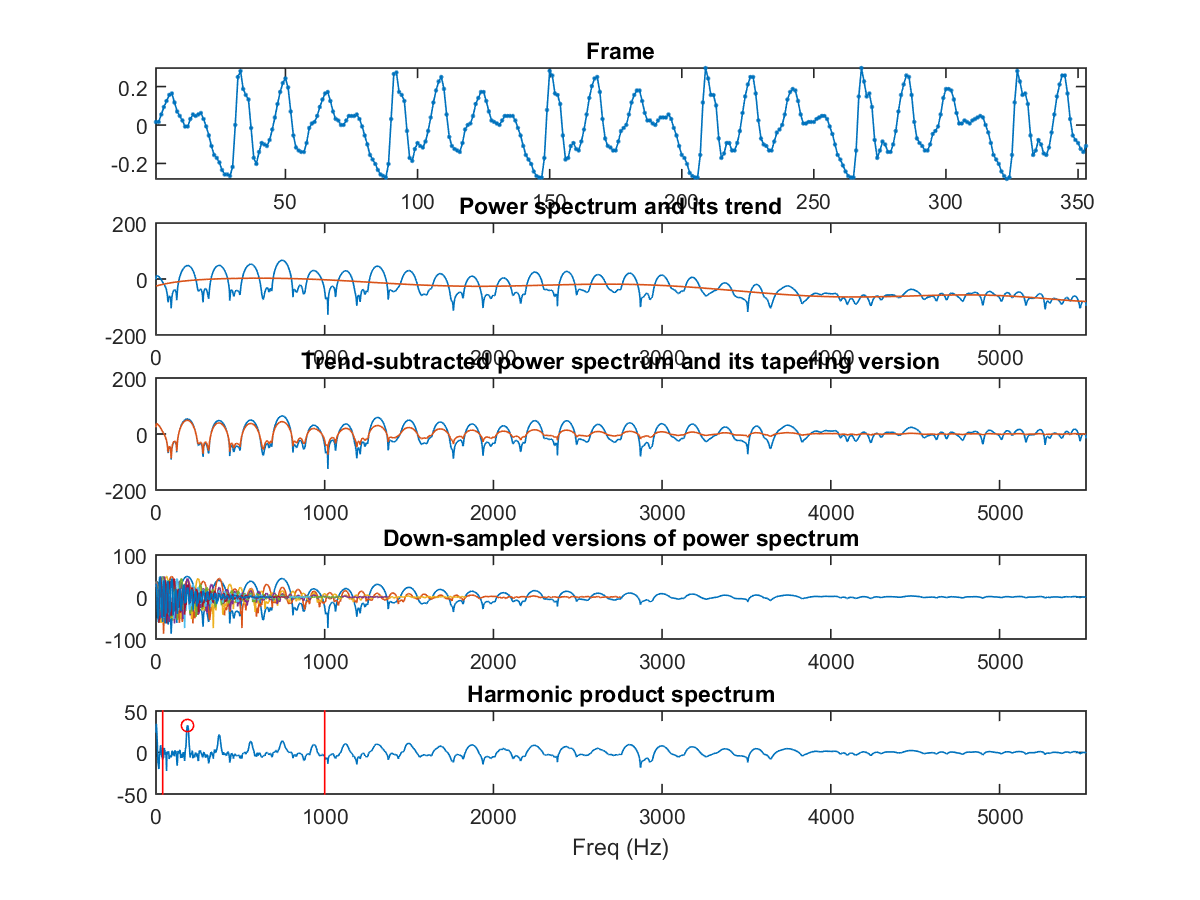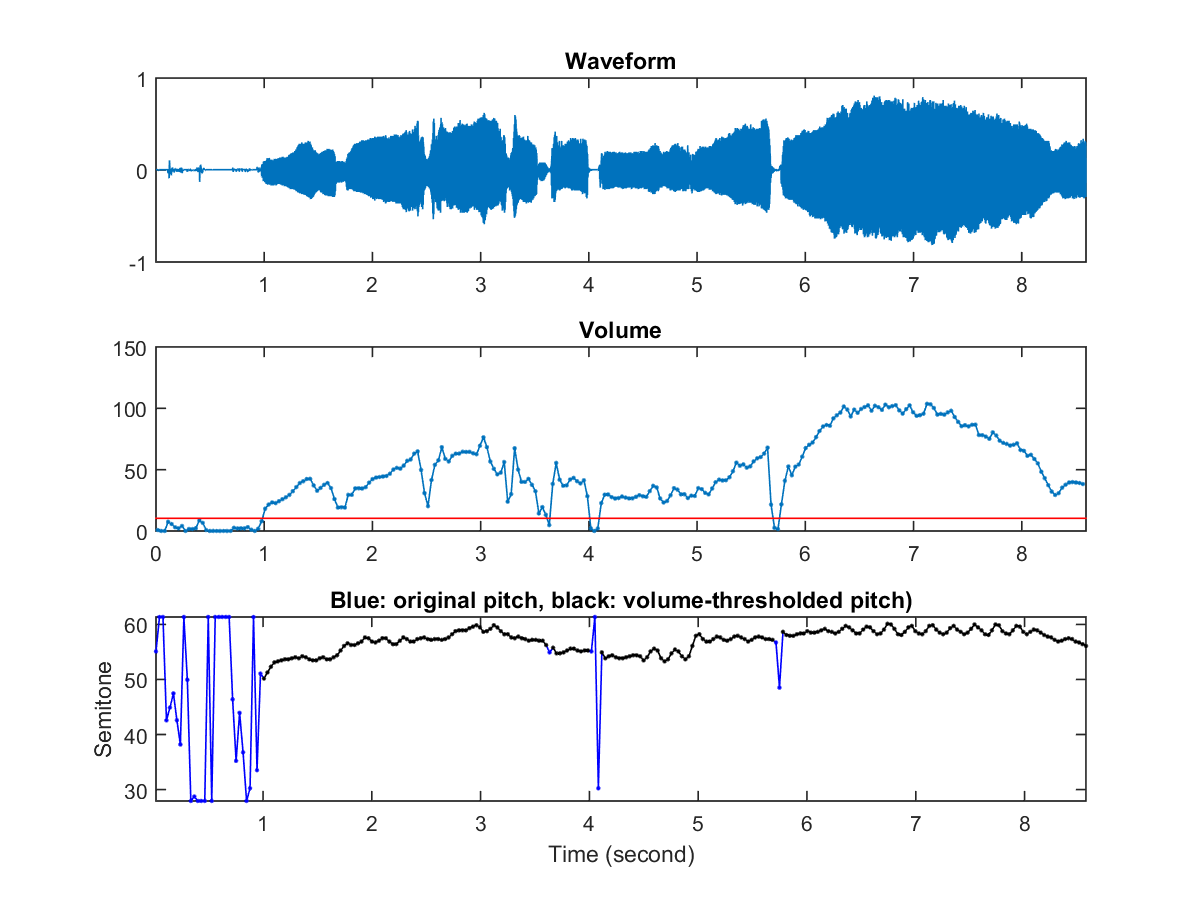## 7-5 Frequency-domain: HPS

• Harmonic product spectrum (HPS)
• Cepstrum methodExample 1: frame2hps01.mwaveFile = 'soo.wav'; au=myAudioRead(waveFile); startIndex=15000; frameSize=round(32*au.fs/1000); % 32ms endIndex=startIndex+frameSize-1; frame = au.signal(startIndex:endIndex); zeroPaddedFactor=15; [hps, freq, spec0, spec1, spec2]=frame2hps(frame, au.fs, zeroPaddedFactor, 1); Pitch = 54.222833 semitoneIn the above plot:
1. Subplot 1 is the waveform of the frame.
2. Subplot 2 shows the power spectrum and its trend estimated by a 20-order polynomial.
3. Subplot 3 shows the trend-subtracted power spectrum and its tapering version.
4. Subplot 4 shows the components of HPS, which are of down-sampled versions of tapering power spectrum.
5. Subplot 5 shows the HPS and its maximum within a reasonable range for human's pitch.

HPS 的特性說明如下：

• HPS 所得到的音高解析度並不高，以上述範例而言，若不進行補零（Zero Padding），則無論最高點的位置為何，音高都會是 11025/256 = 43.07 Hz z的倍數。若需要提高解析度，我們可以將音框進行補零，此時在頻譜就會有較高的解析度，得到的音高解析度也會提高。在上述範例中，我們進行補零直到音框長度為 16*256 = 4096，所得到的頻率解析度是 fs/4096 = 11025/4096 = 2.69 Hz。
• 因為頻譜容易受到共振峰的影響，所以有時候 HPS 得到的最大值並非對應於正確音高。因此在上述範例中，我們先以多項式來預估頻譜的走勢，再將此走勢從頻譜中扣除（以降低共振峰的影響），同時再讓頻譜值隨頻率變大而漸小（以降低高頻部分的影響），再來進行 HPS，得到的效果比較理想。
The following example uses HPS for pitch tracking:

Example 2: ptByHps01.mwaveFile='soo.wav'; opt=pitchTrackBasic('defaultOpt'); opt.frame2pitchOpt.pdf='hps'; showPlot=1; pitch=pitchTrackBasic(waveFile, opt, showPlot);Audio Signal Processing and Recognition (音訊處理與辨識)